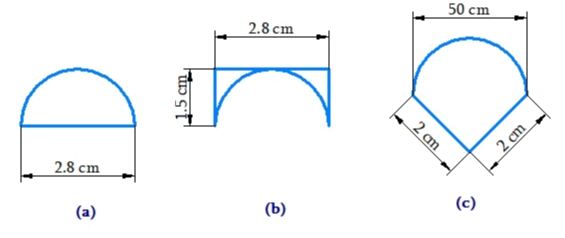# Ex.11.1 Q5 Mensuration Solutions - NCERT Maths Class 8

Go back to  'Ex.11.1'

## Question

An ant is moving around a few food pieces of different shapes scattered on the floor. For which food-piece would the ant have to take a longer round? Remember, circumference of a circle can be obtained by using the expression $$c = 2{\rm{\pi }}r$$ , where $$r$$ is the radius of the circle.Video Solution
Mensuration
Ex 11.1 | Question 5

## Text Solution

What is Known?

Shape and dimensions of various food pieces.

What is unknown?

Perimeter of different shaped food pieces.

Reasoning:

Hence, we will calculate perimeter of different shaped food piece. Larger the perimeter, longer will be the path to take round.

Steps:

(a) Radius of the semicircle part

\begin{align} = \left( {\frac{{2.8}}{2}} \right)\,{\rm{cm}} = 1.4\,{\rm{cm}}\end{align}

Perimeter of the circle $$= 2{{ \pi }}r$$

$$\therefore$$ Perimeter of the semicircle = $$\pi r$$

The perimeter of the food piece

\begin{align}&= 2.8\,{\rm{cm}} + \pi r\\ &= 2.8\,{\rm{cm}} + \left( {\frac{{22}}{{\not7}} \times{{\not\!\!\!\!{1.4}}^{0.2}}} \right)\,{\rm{cm}}\\ &= {\rm{ }}2.8\,{\rm{cm }} + {\rm{ }}4.4\,{\rm{cm}}\\ &= 7.2\,{\rm{cm}}\end{align}

\begin{align} = \frac{{2.8}}{2} = 1.4\,\,{\rm{cm}}\end{align}

Perimeter of the food piece

\begin{align}&= 1.5\,{\rm{cm}} + 2.8\,{\rm{cm}} + 1.5\,{\rm{cm}} + \pi r\\&= 5.8\,{\rm{cm}} + \left( {\frac{{22}}{{\not7}} \times \not\!\!\!\!{{1.4}^{0.2}}} \right){\rm{cm}}\\&= 5.8\,\,{\rm{cm}} + 4.4\,\,c{\rm{m}}\\& = 10.2\,\,{\rm{cm}}\end{align}

(c). Radius of the food piece

\begin{align} = \frac{{2.8}}{2} = 1.4\,\,{\rm{cm}}\end{align}

Perimeter of the food piece

\begin{align}&= 2{\rm{cm}} + \pi r + 2{\rm{cm}}\\& = 4\,{\rm{cm}} + \left( {\frac{{22}}{{\not7}} \times \not\!\!\!\!{{1.4}^{0.2}}} \right){\rm{cm}}\\& = 4\,\,{\rm{cm}} + 4.4\,\,c{\rm{m}}\\& = {\rm{8}}{\rm{.4}}\,\,{\rm{cm}}\end{align}

Thus, the ant will have to take a longer round for food piece (b) because the perimeter of the figure given in (b) is the greatest among all.

Learn from the best math teachers and top your exams

• Live one on one classroom and doubt clearing
• Practice worksheets in and after class for conceptual clarity
• Personalized curriculum to keep up with school